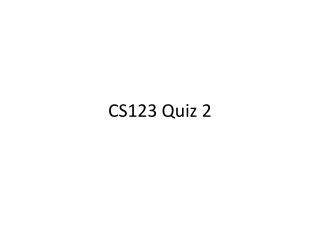DownloadDownload PresentationCS123 Quiz 2

# CS123 Quiz 2

Download Presentation## CS123 Quiz 2

- - - - - - - - - - - - - - - - - - - - - - - - - - - E N D - - - - - - - - - - - - - - - - - - - - - - - - - - -
##### Presentation Transcript

1. CS123 Quiz 2

2. Question 5 • HVAC system • When t <=74, the fan rate is 2360; • When t >= 86, the fan rate is 3870; • (a) What is the formula when 74 < t < 86? • Multiple ways to solve this problem • The easiest way is to use solve function to solve linear equations of 2 unknowns • solve({74*a+b=2360, 86*a+b=3870}, [a,b]);

3. (b) What is the fan rate when the t is 75? • Since 75 is between 74 and 86, we can define a function or procedure based on the formula from (a) • a and b are known at this point • use evalf() to approximate the result to a float point number • To define a procedure • fanRate:=proc(t) return evalf(a*t+b); end; • Or, to define a function • fanRate:=(t)->evalf(a*t+b);

4. (c) Given a decimal point number, we need to round it up to the nearest multiple of then • For example: 2380.8 => 2390 • In order to do so, we need to divide it by 10 • For example: 2380.8/10 => 238.08 • Then take its ceiling, to round it up(or down) to the nearest integer • For example: ceil(238.08) => 239; or floor(238.08)=>238; • At last, to multiple it by 10 • For example: 239 * 10 => 2390

5. (d) use map function to easily apply the procedure you defined in (3) to the list of temperatures. • Assume tempList is your list of temperatures • map(C, tempList);

6. (e) • You already have the procedure definition so far, but you need to put the value of a and b back to the procedure, if you claim them before the procedure in (c) as I did. • For example: • result:=ceil((755/6*t-20855/3)/10)*10; (round up) • Or floor((755/6*t-20855/3)/10)*10; (round down)